# Comparing Frequencies with an Oscilloscope

This article describes a method for the very precise measurement of a frequency difference using an oscilloscope with multiple channels.

The following video shows the display of an oscilloscope whose four channels are connected to four different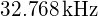Temperature Compensated Crystal Oscillators (TCXOs).

As seen in the above video, four different waveforms originating form the oscillators are displayed on the oscilloscope screen. The TCXOs are very accurate and are rated at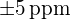, so their frequencies are very close to each other. The waveform on channel 4 (dark blue) has been used as the trigger source, this waveform shall represent the reference frequency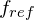. The waveforms on channels 1, 2 and 3 (yellow, light blue and purple) are slowly drifting relative to the reference waveform. Thus, these waveforms have ever so slightly different frequencies whose difference we are about to determine.

In order to determine the exact frequency difference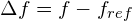, we need to first measure how fast the corresponding waveform is scrolling relative to the reference waveform. For this purpose, we shall define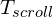as the time duration in seconds that is required for the waveform to move by one whole period relative to one of the fixed vertical lines on the oscilloscope screen.can be determined by visually observing the waveform and measuring one period scroll duration using a stopwatch.

If a waveform is scrolling towards the left side relative to the reference waveform, then its frequency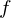is larger thanandshall have a positive sign. The opposite is true if the waveform is scrolling towards the right side, then its frequencyis smaller thanandshall have a negative sign.

Wheneverelapses, the measured waveform will advance or retard by one full period duration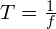relative to the reference waveform.

For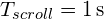, the measured waveform will advance by exactly one period per second. Consequently, the frequency difference relative towould be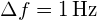. This behaviour can be generalised by the following formula: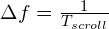The difference in parts per million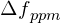can be calculated as follows: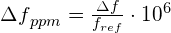The TCXOs measured in the above setup have been used for increasing the accuracy of a classic Casio digital wrist watch. Hence, it is of our interest to calculate the particular oscillator’s drift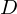in seconds per day. This can be done using the following formula: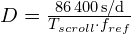Taking the purple waveform on channel 3 as an example, we can measure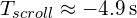. This would yield to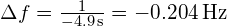.

For the above example,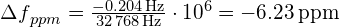and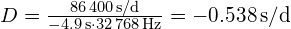.

I hope that you found this article useful. Please be welcome post your feedback and comments below.